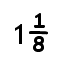Home Math Calculators Math  Flashcards Math Games Math  Homework Math Lessons Math Terms Math Worksheets Math Tools
You Are In Math Glossary of Terms

# Mixed Number

A mixed number is a number that is written as a whole number plus a fraction.

Example: